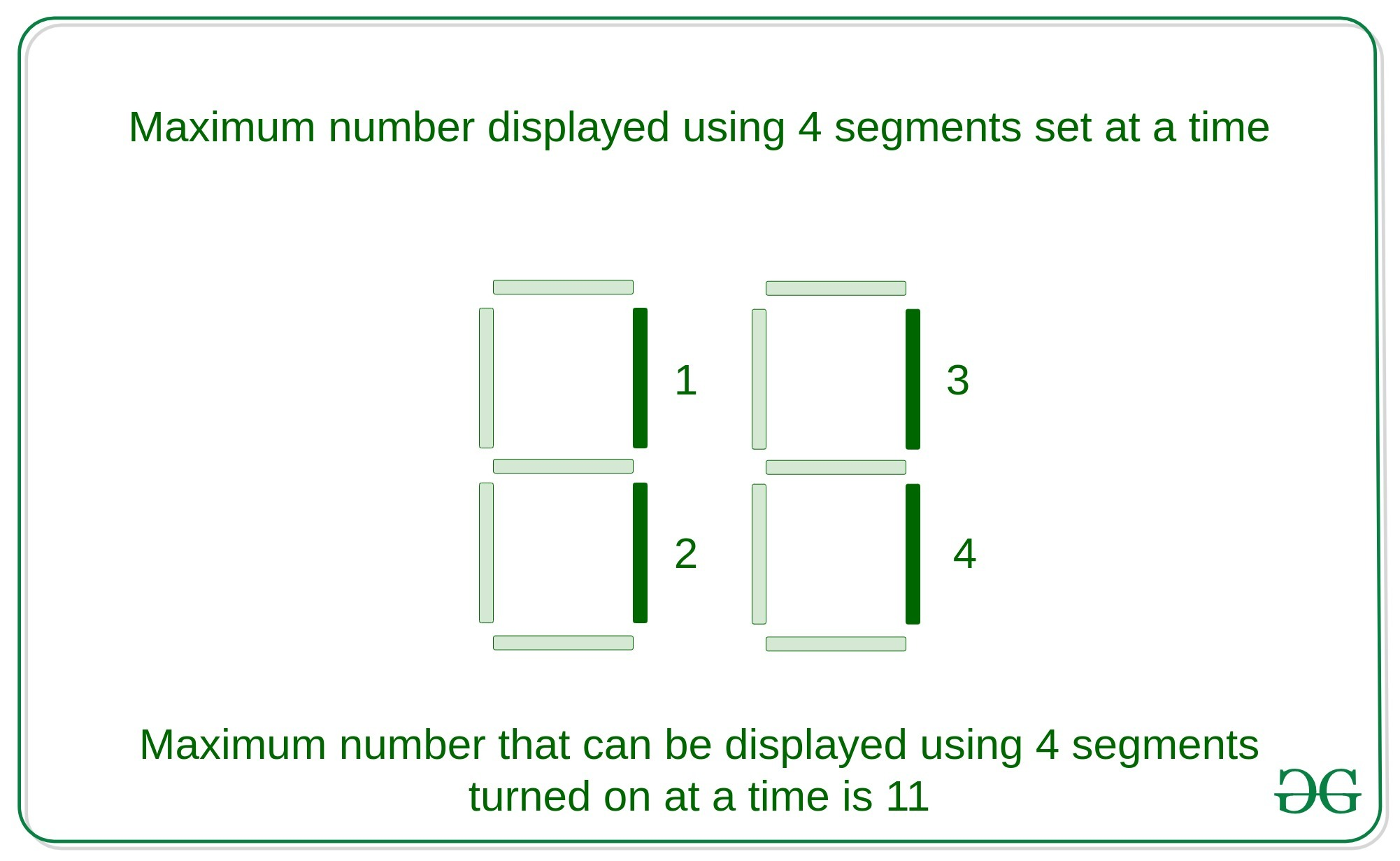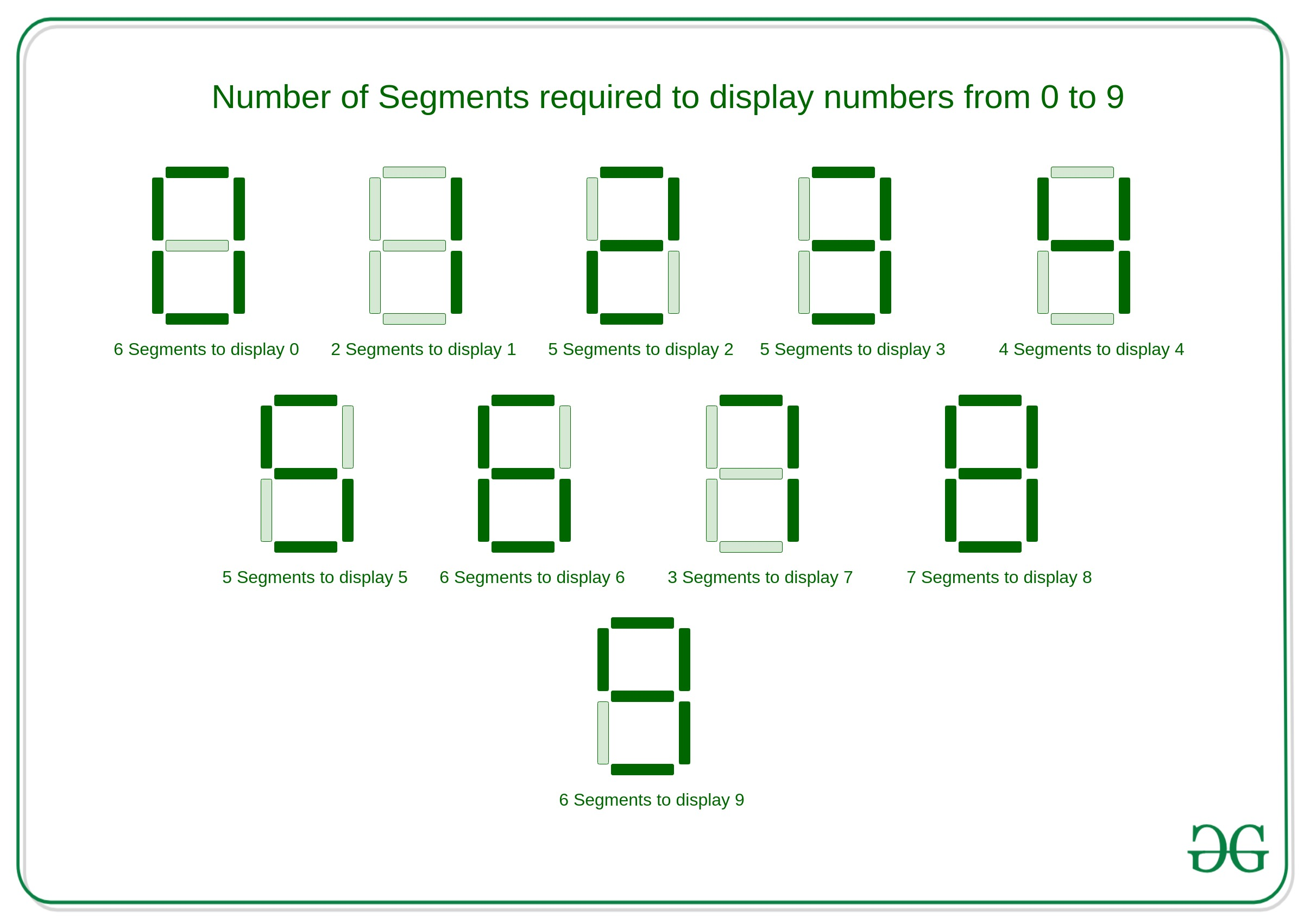Maximum number on 7-segment display using N segments : Recursive

• Last Updated : 10 Jun, 2021

Given an integer N, the task is to find the largest number that can be shown with the help of N segments using any number of 7 segment displays.
Examples:

Input: N = 4
Output: 11
Explanation:
Largest number that can be displayed with the help of 4 segments using 2 seven segment displays turned is 11.Input: N = 7
Output: 711
Explanation:
Largest number that can be displayed by turning on seven segments is 711 with the help of 3 segments display set.

Approach:
The key observation in seven segment display is to turn on any number from 0 to 9 takes certain amounts of segments, which is described below:If the problem is observed carefully, then the number N can be of two types that is even or odd and each of them should be solved separately as follows:

• For Even: As in the above image, There are 6 numbers that can be displayed using even number of segments which is

0 - 6
1 - 2
2 - 5
4 - 4
6 - 6
9 - 6
• As it is observed number 1 uses the minimum count of segments to display a digit. Then, even the number of segments can be displayed using 1 with 2 counts of segments in each digit.

• For Odd: As in the above image, there are 5 numbers that can be displayed using an odd number of segments which is

3 - 5
5 - 5
7 - 3
8 - 7
• As it is observed number 7 uses the minimum number of odd segments to display a digit. Then an odd number of segments can be displayed using 7 with 3 counts of segments in each digit.

Algorithm:

• If the given number N is 0 or 1, then any number cannot be displayed with this much of bits.
• If the given number N is odd then the most significant digit will be 7 and the rest of the digits can be displayed with the help of the (N – 3) segments because to display 7 it takes 3 segments.
• If given number N is even then the most significant digit will be 1 and the rest of the digits can be displayed with the help of the (N – 2) segments because to display 1, it takes 2 segments only.
• The number N is processed digit by digit recursively.

Explanation with Example:
Given number N be – 11

Then, the largest number will be 71111.
Below is the implementation of the above approach:

C++

 // C++ implementation to find the// maximum number that can be// using the N segments in// N segments display #include using namespace std; // Function to find the maximum// number that can be displayed// using the N segmentsvoid segments(int n){    // Condition to check base case    if (n == 1 || n == 0) {        return;    }    // Condition to check if the    // number is even    if (n % 2 == 0) {        cout << "1";        segments(n - 2);    }         // Condition to check if the    // number is odd    else if (n % 2 == 1) {         cout << "7";        segments(n - 3);    }} // Driver Codeint main(){    int n;    n = 11;    segments(n);    return 0;}

Java

 // Java implementation to find the// maximum number that can be// using the N segments in// N segments displayclass GFG {         // Function to find the maximum    // number that can be displayed    // using the N segments    static void segments(int n)    {        // Condition to check base case        if (n == 1 || n == 0) {            return;        }        // Condition to check if the        // number is even        if (n % 2 == 0) {            System.out.print("1");            segments(n - 2);        }                 // Condition to check if the        // number is odd        else if (n % 2 == 1) {                 System.out.print("7");            segments(n - 3);        }    }         // Driver Code    public static void main (String[] args)    {        int n;        n = 11;        segments(n);    }} // This code is contributed by AnkitRai01

Python3

 # Python3 implementation to find the# maximum number that can be# using the N segments in# N segments display # Function to find the maximum# number that can be displayed# using the N segmentsdef segments(n) :     # Condition to check base case    if (n == 1 or n == 0) :        return;         # Condition to check if the    # number is even    if (n % 2 == 0) :        print("1",end="");        segments(n - 2);         # Condition to check if the    # number is odd    elif (n % 2 == 1) :         print("7",end="");        segments(n - 3); # Driver Codeif __name__ == "__main__" :     n = 11;    segments(n); # This code is contributed by AnkitRai01

C#

 // C# implementation to find the// maximum number that can be// using the N segments in// N segments displayusing System; class GFG {         // Function to find the maximum    // number that can be displayed    // using the N segments    static void segments(int n)    {        // Condition to check base case        if (n == 1 || n == 0) {            return;        }        // Condition to check if the        // number is even        if (n % 2 == 0) {            Console.Write("1");            segments(n - 2);        }                 // Condition to check if the        // number is odd        else if (n % 2 == 1) {                 Console.Write("7");            segments(n - 3);        }    }         // Driver Code    public static void Main()    {        int n;        n = 11;        segments(n);    }} // This code is contributed by AnkitRai01

Javascript


Output:
71111

Performance Analysis:

• Time Complexity: As in the above approach, there is recursive call which takes O(N) time in worst case, Hence the Time Complexity will be O(N).
• Auxiliary Space Complexity: As in the above approach, taking consideration of the stack space used in recursive call then the auxiliary space complexity will be O(N)

My Personal Notes arrow_drop_up